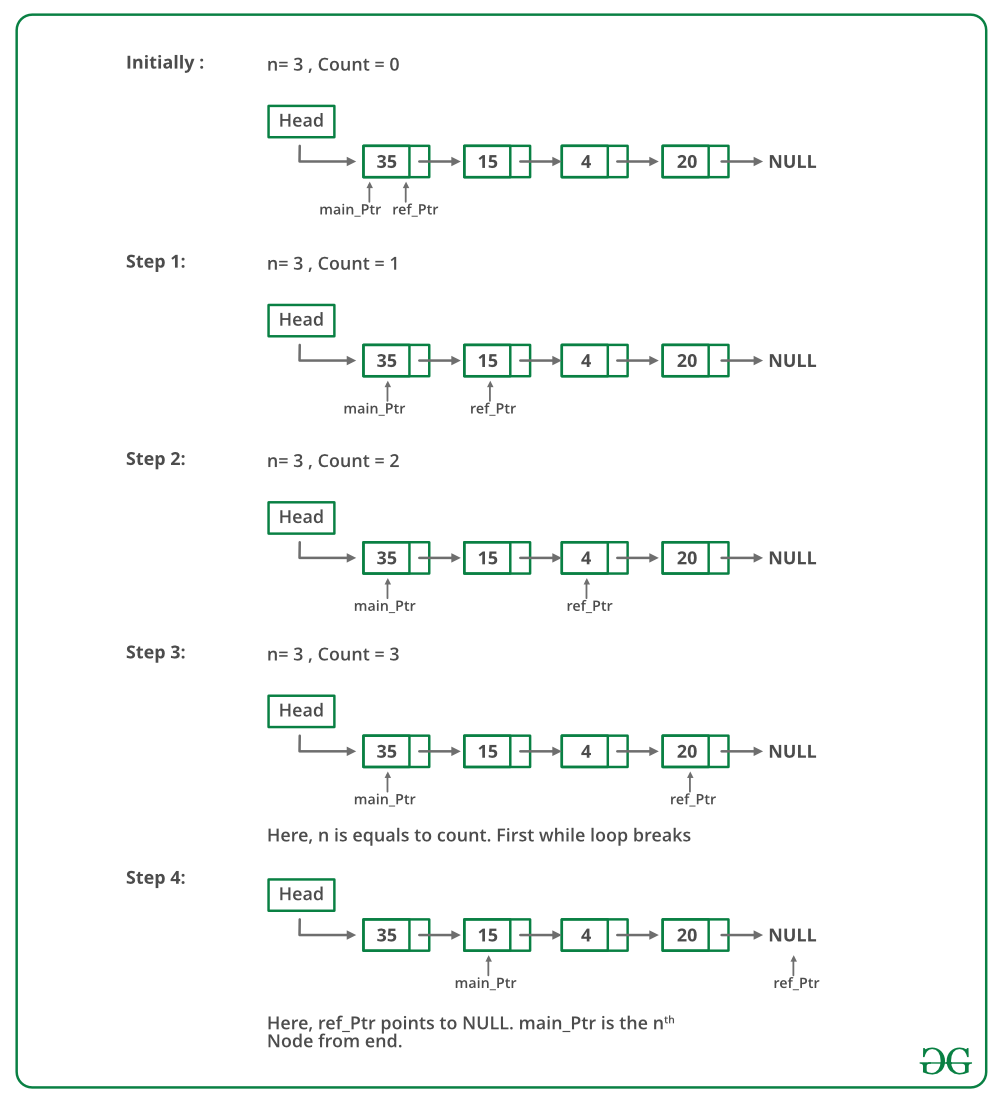GeeksforGeeks App
Open AppBrowser
Continue

# Java Program For Printing Nth Node From The End Of A Linked List

Given a Linked List and a number n, write a function that returns the value at the n’th node from the end of the Linked List.
For example, if the input is below the list and n = 3, then the output is “B”.### Method 1 (Use length of linked list)

1) Calculate the length of the Linked List. Let the length be len.
2) Print the (len – n + 1)th node from the beginning of the Linked List.

### Double pointer concept:

First pointer is used to store the address of the variable and the second pointer is used to store the address of the first pointer. If we wish to change the value of a variable by a function, we pass a pointer to it. And if we wish to change the value of a pointer (i. e., it should start pointing to something else), we pass the pointer to a pointer.

Below is the implementation of the above approach:

## Java

 `// Simple Java program to find n'th node from end of linked list``class` `LinkedList {``    ``Node head; ``// head of the list` `    ``/* Linked List node */``    ``class` `Node {``        ``int` `data;``        ``Node next;``        ``Node(``int` `d)``        ``{``            ``data = d;``            ``next = ``null``;``        ``}``    ``}` `    ``/* Function to get the nth node from the last of a``       ``linked list */``    ``void` `printNthFromLast(``int` `n)``    ``{``        ``int` `len = ``0``;``        ``Node temp = head;` `        ``// 1) count the number of nodes in Linked List``        ``while` `(temp != ``null``) {``            ``temp = temp.next;``            ``len++;``        ``}` `        ``// check if value of n is not more than length of``        ``// the linked list``        ``if` `(len < n)``            ``return``;` `        ``temp = head;` `        ``// 2) get the (len-n+1)th node from the beginning``        ``for` `(``int` `i = ``1``; i < len - n + ``1``; i++)``            ``temp = temp.next;` `        ``System.out.println(temp.data);``    ``}` `    ``/* Inserts a new Node at front of the list. */``    ``public` `void` `push(``int` `new_data)``    ``{``        ``/* 1 & 2: Allocate the Node &``                  ``Put in the data*/``        ``Node new_node = ``new` `Node(new_data);` `        ``/* 3. Make next of new Node as head */``        ``new_node.next = head;` `        ``/* 4. Move the head to point to new Node */``        ``head = new_node;``    ``}` `    ``/*Driver program to test above methods */``    ``public` `static` `void` `main(String[] args)``    ``{``        ``LinkedList llist = ``new` `LinkedList();``        ``llist.push(``20``);``        ``llist.push(``4``);``        ``llist.push(``15``);``        ``llist.push(``35``);` `        ``llist.printNthFromLast(``4``);``    ``}``} ``// This code is contributed by Rajat Mishra`

Output

`35`

Time complexity: O(n)

Auxiliary Space: O(1) since using constant space

Following is a recursive C code for the same method. Thanks to Anuj Bansal for providing the following code.

## Java

 `static` `void` `printNthFromLast(Node head, ``int` `n)``{``    ``static` `int` `i = ``0``;` `    ``if` `(head == ``null``)``        ``return``;``    ``printNthFromLast(head.next, n);` `    ``if` `(++i == n)``        ``System.out.print(head.data);``}` `// This code is contributed by rutvik_56.`

Time Complexity: O(n) where n is the length of linked list.

Auxiliary Space: O(n) for call stack because using recursion.

### Method 2 (Use two pointers)

Maintain two pointers – the reference pointer and the main pointer. Initialize both reference and main pointers to head. First, move the reference pointer to n nodes from head. Now move both pointers one by one until the reference pointer reaches the end. Now the main pointer will point to nth node from the end. Return the main pointer.

Below image is a dry run of the above approach:## Java

 `// Java program to find n'th``// node from end using slow and``// fast pointers` `class` `LinkedList {``    ``Node head; ``// head of the list` `    ``/* Linked List node */``    ``class` `Node {``        ``int` `data;``        ``Node next;``        ``Node(``int` `d)``        ``{``            ``data = d;``            ``next = ``null``;``        ``}``    ``}` `    ``/* Function to get the``      ``nth node from end of list */``    ``void` `printNthFromLast(``int` `n)``    ``{``        ``Node main_ptr = head;``        ``Node ref_ptr = head;` `        ``int` `count = ``0``;``        ``if` `(head != ``null``) {``            ``while` `(count < n) {``                ``if` `(ref_ptr == ``null``) {``                    ``System.out.println(``                        ``n + ``" is greater than the no "``                        ``+ ``" of nodes in the list"``);``                    ``return``;``                ``}``                ``ref_ptr = ref_ptr.next;``                ``count++;``            ``}` `            ``if` `(ref_ptr == ``null``) {` `                ``if` `(head != ``null``)``                    ``System.out.println(``"Node no. "` `+ n``                                       ``+ ``" from last is "``                                       ``+ head.data);``            ``}``            ``else` `{` `                ``while` `(ref_ptr != ``null``) {``                    ``main_ptr = main_ptr.next;``                    ``ref_ptr = ref_ptr.next;``                ``}``                ``System.out.println(``"Node no. "` `+ n``                                   ``+ ``" from last is "``                                   ``+ main_ptr.data);``            ``}``        ``}``    ``}` `    ``/* Inserts a new Node at front of the list. */``    ``public` `void` `push(``int` `new_data)``    ``{``        ``/* 1 & 2: Allocate the Node &``                  ``Put in the data*/``        ``Node new_node = ``new` `Node(new_data);` `        ``/* 3. Make next of new Node as head */``        ``new_node.next = head;` `        ``/* 4. Move the head to point to new Node */``        ``head = new_node;``    ``}` `    ``/*Driver program to test above methods */``    ``public` `static` `void` `main(String[] args)``    ``{``        ``LinkedList llist = ``new` `LinkedList();``        ``llist.push(``20``);``        ``llist.push(``4``);``        ``llist.push(``15``);``        ``llist.push(``35``);` `        ``llist.printNthFromLast(``4``);``    ``}``}``// This code is contributed by Rajat Mishra`

Output

`Node no. 4 from last is 35 `

Time Complexity: O(n) where n is the length of linked list.

Auxiliary Space: O(1) using constant space.

Please refer complete article on Program for n’th node from the end of a Linked List for more details.

My Personal Notes arrow_drop_up